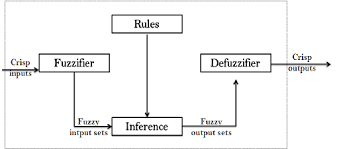# Fuzzy control logic for steam turbine### What is fuzzy control?

A simple fuzzy controller is designed for a system. Unlike observer-based approaches, no mathematical model is used. Fuzzy controller uses Fuzzy control logic. The control strategy is generated by formulating the tasks which need to be carried out to keep the system in an upright position.

The controller consists of the following three parts:

• Fuzzifier
• Inference network
• DefuzzifierThe fuzzifier transforms a crisp value into a fuzzy value. Mathematically this can be denoted as:where n is the number of fuzzy sets influenced by the crisp value x, x = [-L, L] and u(x), I [0, 1] is the compatibility degree of the ith fuzzy set. The a1 ai [0, 1]V/ i describe the fuzzification operator which is, in this case, the product
of the compatibility degree and the membership function. The inference network then provides the fuzzy output value u(f). The fuzzy output u(f) is the accumulated output of all the rules in the inference network.

### Fuzzy controller for a steam turbine:

In this feasibility study a fuzzy logic speed controller has to be applied to a steam turbine. The efficiency of the fuzzy control was compared with that of conventional PID control. As a basis for this comparison we used a mathematical model of the turbine which has been well validated on a real turbine, in connection with the design of the PID control. A simple fuzzy controller was designed for this model.

To assess the resulting fuzzy control we studied by simulation the step response of the speed due to a sudden load reduction of 100 %, as well as the robustness with respect to parameter variations of the turbine.

The control task can be divided into two parts:

1. Speed control of the turbine;
2. Pressure control of the turbine.

In the speed control of a steam turbine the following two tasks are of primary interest:

• Run-up of the turbine to nominal speed;
• Catch a sudden load reduction at nominal speed.

To investigate the second task, we use both the dynamic model of the steam turbine as well as the PID control concept. It can be seen from Figure, that the overshoot of the fuzzy-controlled turbine, due to a sudden load reduction of the turbine, is much less than the overshoot of the PID-controlled turbine. The simulation results show that even a relatively simple fuzzy logic control algorithm can produce better results than a PID controller.Scroll to Top